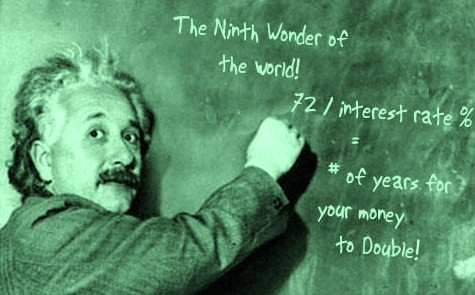You may have heard the saying ‘If it sounds too good to be true, it probably isn’t true’. But how do you work out what could be too good to be true?

Start with the rate of return you have been offered. Most investments illustrate their rates of return using percentages. While that’s perfectly reasonable, research suggests that many people have trouble working out percentages, especially in their heads.

To determine how many years for your capital to double, you bring to mind the Rule of 72, which tells you to always divide the capital by the interest, and the result is in how many years it will be doubled. This is simpler than it seems. Before calculators or spreadsheets, investors used the trusty old ‘Rule of 72’.

How the Rule of 72 works

Suppose you were offered an investment with a return of 10% per year and you reinvested all your returns. How many years would it take to double the value of your original investment?

The Rule of 72 tells you: divide 72 by the annual rate of return to get the number of years it will take to double your money.

So for 10% return per year: 72 divided by 10 = 7.2 years for your original investment to double.

If you get a 20% per year return, it will take a shade over 3½ years to double your money (72 divided by 20 = 3.6 years). Similarly, if you get only 3% per year you will have to wait 24 years (72 divided by 3 = 24 years).

The Rule of 72 is not absolutely precise, but it gives you a practical estimate that you can work out in your head. By using the Rule of 72, you can find out if an investment return really is ‘too good to be true’, and avoid being taken in by scams.

The Rule of 72 also forecasts the value of money

In finance, the Rule of 72 is a method for estimating an investment’s doubling time or halving time. These rules apply to exponential growth and decay respectively, and are therefore used for compound interest as opposed to simple interest calculations.

To determine the time it takes for the value of money to halve at a given rate, divide the rule quantity by that rate.

To determine the time for money’s buying power to halve, financiers simply divide the “rule-quantity” by the inflation rate. Thus at 3.5% inflation using the rule of 72, it should take approximately 72/3.5 = 20.571 years for the value of a dollar to halve.